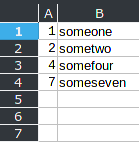# How to distribute rows in sheet using column as row number

I would like to import data or ‘sort’ data in a sheet using a column value as row number.

esample:

my data is

``````1, someone
2, sometwo
4, somefour
7, someseven
``````

and I need to automatically distribute in a sheet leaving empty lines on row 3, 5 and 6
is it possible? Thanks

Hello

one solution:

• Import your data into column `A` and `B` this way:• Enter formula `=IFNA(OFFSET(\$B\$1;MATCH(ROW();\$A\$1:\$A\$4;0)-1;0;1;1);"")` into `C1`

• Drag the formula down to row which equals the maximum number in column `A`, which is `7` in this example. In other words: Drag down to cell `C7`

• Optional Steps

1. Select column `C` and `Data -> Calculate -> Formula to Value`
2. Delete columns `A` and `B`

Hope that helps

Thanks, it works.

hallo

``````=IFNA(INDEX(B:B;MATCH(ROW(D1:D20);A:A;0));"")
``````

of course with `[x] Matrix` option in Formula-Assistent